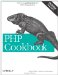# Recipe 3.6. Finding the Difference of Two Dates with Julian Days

#### 3.6.1. Problem

You want to find the difference of two dates measured by what a clock would say, not the actual elapsed time.

#### 3.6.2. Solution

Use gregoriantojd( ) to get the Julian day for a set of date parts and then subtract one Julian day from the other to find the date difference. Then, convert the time parts to seconds and subtract one from the other to find the time difference. If the time difference is less than 0, decrease the date difference by one and adjust the time difference to apply to the previous day. Example 3-15 shows how to do this.

##### Finding date differences with Julian days

 ``

#### 3.6.3. Discussion

Finding differences with Julian days lets you operate outside the range of epoch seconds and also accounts for DST differences.

Example 3-16 does the calculation with date parts from arrays.

##### Calculating difference with arrays of date parts

 ``

Example 3-16 prints:

`The two dates have 128 weeks, 6 days, 15 hours, 3 minutes, and 45 seconds between them.`

This method produces a time difference based on clock time, which is why the result shows an hour more of difference than in Recipe 3.5. May 10 is during DST, and November 20 is during standard time.

The function gregoriantojd( ) is part of PHP's calendar extension, and so is available only if that extension is loaded.

Recipe 3.5 to find the difference between two dates in elapsed time; Recipe 3.10 for adding and subtracting from a date; documentation on gregoriantojd( ) at http://www.php.net/gregoriantojd; an overview of the Julian day system is at http://tycho.usno.navy.mil/mjd.html.PHP Cookbook: Solutions and Examples for PHP Programmers
ISBN: 0596101015
EAN: 2147483647
Year: 2006
Pages: 445

Similar book on Amazon